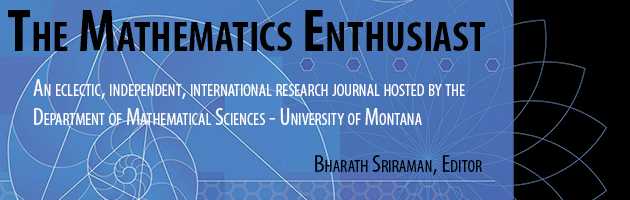•
•3

2

#### Abstract

According to L. Wittgenstein, the meaning of a mathematical object is to be grounded upon its use. In this paper we consider Robinson theory Q, the subtheory of firstorder Peano Arithmetic PA; some theorems and conjectures can be interpreted over one model of Q given by a universe of polynomials; with respect to nonconstant polynomials some proofs by elementary methods are given and compared with corresponding results in the standard model of PA. We conclude that the creative power of the language can be pointed out in how the language itself is embedded into the rest of human activities, and this is an important track to follow for researchers in mathematics education.

146

156

COinS